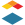# Canvas Outcomes: New and Updated Proficiency Calculations Coming SoonInstructure
0 0 310When speaking to Canvas users, I often hear about the need for more flexibility in grading, especially for those using more non-traditional methods outside of those that rely heavily on the traditional use of points, percentages, and letter grades.  To better support the various philosophies of our users when it comes to proficiency-based grading practices (you may also know this as outcomes-, mastery-, competency-based, etc), we’re updating the proficiency calculation methods available in Canvas LMS.

Based on feedback from many institutions and educators that use our Learning Mastery Gradebook and Outcomes, the current Decaying Average calculation does not reflect their practices or the traditionally accepted formula for this method. This update adds a new Decaying Average option with a formula based on the traditional decaying average calculation. This also makes calculation methods consistent across Canvas and Mastery Connect for those using both products in our Instructure Learning Platform. For those using the current calculation, we will be renaming the currently available ‘Decaying Average’ to ‘Weighted Average’ to better reflect how the formula works.

Administrators and Teachers with appropriate permissions can select the new ‘Decaying Average’ when setting up proficiency calculations.  Depending on your instance settings, this may apply at the individual outcome, course, or account level.

This update ensures that all instances currently using the existing ‘Decaying Average’ - now ‘Weighted Average’ - are updated to reflect the new name and will continue utilizing its calculation method.

### Let’s take a look at how these calculations work.

#### Decaying Average

The Decaying Average proficiency calculation uses a formula that determines proficiency based on students' average scores, giving more weight to the most recent scored.

To determine the weight given to the most recent score, the formula uses a decay rate. The higher the formula's decay rate, the more heavily the most recent assessment is weighted.

By default, the decay weight is set at 65%. However, users can adjust the rate to any percentage between 50% and 99%.

To show an example, between two assessments, the most recent assessment gets 65% weight, and the first gets 35%. For each additional assessment, the sum of the previous score calculations decay by an additional 35%. The math behind the 65% decaying average works like this; let's say you have four assessments that receive the following scores: 1, 2, 3, 4 (this last one being the most recent):

(1 × .35) + (2 × .65) = X

(X × .35) + (3 × .65) = Y

(Y × .35) + (4 × .65) = Z  (this being the current standard score; 3.48)

#### Weighted Average

The previous Canvas method, now Weighted Average, uses a simplified approach where Canvas calculates the average of all prior assessments and applies the value as a weighted percentage formula.

By default, the Canvas weighted average is set at 65/35, meaning the most recent score is weighted at 65%, and the average of all other scores is weighted at 35%. Again, users can adjust this parameter this time with the option to specify between 1% and 99% for the most recent percentage weight, and prior scores are weighted against the percentage difference.

For example, let’s assume a student has four aligned items with scores of 4, 3, 2, and 5 (scores listed chronologically from oldest to most recent). If the most recent score is set to be weighted at 65% of proficiency, prior scores are weighted at 35%.

Let’s see how this works:

Most recent score: 5

Average of prior scores: (4 + 3 + 2) / 3 = 3

Calculated score: 5 (.65) + 3 (.35) = 3.25 + 1.05 = 4.3

### When will these changes be available?

These updates are currently available and can be tested in your beta instance. You can expect them to be available in production with our 16 September 2023 release.